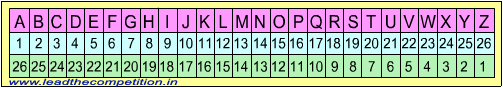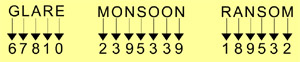#### Test of Reasoning - Questions on Codes1. If BLACKSMITH is coded as CNBELUNKUJ, then CHILDREN will be coded as ?

Explanation: Letters at first, third, fifth, seventh and ninth position in CNBELUNKUJ i.e. C, B, L, N, U are one letter forward of the letters in the same position in BLACKSMITH. Letters at second, fourth, sixth, eighth and tenth position in CNBELUNKUJ i.e. L, C, S, I, H are two letters forward of the letters in the same position in BLACKSMITH. Thus for coding CHILDREN, D, J, E, F follow C, I, D and E and J, N, T, P follow H, L, R and N.

2. If D = 4, DOG = 26, then find the value of ANIMAL= ?

Explanation: The numerical value of the word is the sum of numerical positions of the letters in the alphabet. DOG is 4 + 15 + 7 = 26. Thus ANIMAL is 1 + 14 + 9 + 13 + 1 + 12 = 50.

3. If LIGHT is codes as MJHIU, find the code for NORMAL

Explanation: The letters in MJHIU are one letter forward of the letters in the same position in LIGHT. Thus NORMAL would be OPSNBM.

4. If RATION is written as OXQFLK then LUMBER may be written as _____ .

Explanation:Each letter in OXQFLK is two letters backwards from the corresponding letter in RATION as follows R - QP - O, A - ZY - X, T - SR - Q, I - HG - F, O - NM - L, N - ML - K. Following the same code, LUMBER may be written as IRJYBO.

5. If FRIEND is codes as HTKGPF then REVEAL will be coded as:

Explanation:Each letter in HTKGPF is two letter forwards from the corresponding letter in FRIEND. Thus REVEAL will be coded as TGXGCN.

6. If ‘PAPER’ is written as ‘OZODQ’, how can ‘PENCIL’ be written in that code?

Explanation: Each letter in the given word is replaced by the letter preceding it in the English alphabet. Thus, the first letter P becomes O and the last letter L becomes K. Only option (d) satisfies the condition.

7. In a coding system PEN is written as NZO and BARK as CTSL. How can we write PRANK in that coding system?

Explanation: P -> N, R -> S, A -> T and N -> O. Therefore answer is NSTOL

8. If BROTHER is coded as 2456784, SISTER is coded as 919684, what is the code for ROBBERS?

Explanation: E and R which are common to both the given words and placed at the end correspond to 84. In ROBBERS, ER is placed before S and thus the answer code should contain 84 before the last number. Only option (d) satisfies the condition. The answer is further checked by the numbers 22 which corresponds to BB as B -> 2 in the first example.

9. If MIND becomes KGLB and ARGUE becomes YPESC then what will DIAGRAM be in that code?

Explanation: Each letter in the given word is replaced with the letter which is 2 places behind it in the English alphabet. Thus M becomes K leaving L in between. By the same logic, we get BGYEPYK for DIAGRAM.

10. If GLARE is coded as 67810 and MONSOON as 2395339 then how can RANSOM be coded?

Explanation:Answer (d).11. In a certain code the words COME AT ONCE were written as XLNVZGLMXV, in the same code which of the following would be ‘OK’?

Explanation:Answer (b). The letters are replaced by corresponding letters in the same place but in the reverse order. Thus A which is the first letter is replaced by Z which is the last letter. The 3rd letter C is replaced by X which is 3rd from the other end. See figure at the top of the page for guidance

12. If STUDENT is coded as TSVCFMU, which word would be coded as TGBBLKF?

Explanation:Answer (c). The letters in the odd places viz. S, U, E, T are replaced by letters succeeding them i.e. T, V, F, U and the letters in the even places viz. T, D, N are replaced by letters preceding them i.e. S, C, M. Thus T, B, L, F in the question code should be replaced by letters preceding them i.e. S, A, K, E and G, B, K would be replaced by letters succeeding them i.e. H, C, L. The word would thus be SHACKLE

13. If GARDEN is coded as 325764 and WATER as 92165, how can we code the word WARDEN in the same way?

Explanation:Answer (a). The last 5 letters of the word GARDEN and WARDEN are same. Hence look for 25764 in the answer.

14. If the word EARTH be written as QPMZS in coded words, how can HEART be written following the same coding?

Explanation:Answer (c). The first 4 letters of the word EARTH and the last 4 letters of the word HEART are same. So look for QPMZ in the answer.

15. If ‘BE QUICK’ is coded as ‘ZCOSGAI’, then the code of last letter of third word in the sentence I LOVE MY COUNTRY is

Explanation:Answer (a). Each letter in the word has been replaced by a letter 2 places behind in the English alphabet. Thus E -> C, K -> I etc. In the question, the last letter of the third word is Y. The letter which is 2 places behind Y is W.